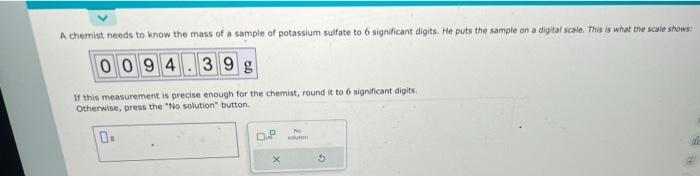Home / Expert Answers / Chemistry / how-do-you-round-to-the-6-significant-digit-nbsp-a-chemist-needs-to-know-the-mass-of-a-sample-of-pa421

# (Solved): How do you round to the 6 significant digit ?  A chemist needs to know the mass of a sample of ...

How do you round to the 6 significant digit ?A chemist needs to know the mass of a sample of potassium sulfate to 6 significant digits. He puts the sample on a digital scale. This is what the scale shows: If this measurement is precise enough for the chemist, round it to 6 significant digits., Otherwise, press the "No sclution" button.

We have an Answer from Expert